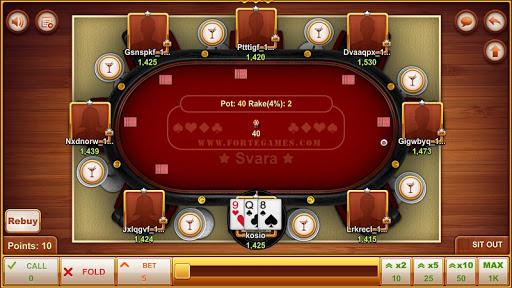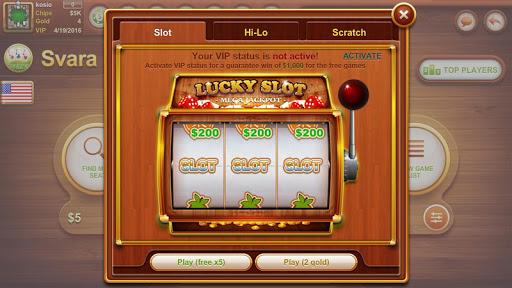# SVARA BY FORTEGAMES ( SVARKA ) 11.0.64 APK

0
70

### Hình ảnh SVARA BY FORTEGAMES ( SVARKA )### Giới thiệu SVARA BY FORTEGAMES ( SVARKA )

Rules of the game Svara ( Svarka )

Svara ( Svarka ) is a card game that is played with a deck of 32 cards – from 7 to Ace . The minimum number of players is two . The total number of combinations in the game is 4960

Rules
————-
Each player is dealt three cards in a clockwise direction . Each card combination has a specific numerical value. Winner is the player with highest points.

The value of the points is calculated by the following rules:
1) Each card from 7 to 9 give 7 to 9 points respectivly.
2 ) The cards 10 , J, Q, K and give 10 points.
3 ) Aces give 11 points .
4 ) The cards of the same color have as many points as their sum . For instance :
Q ♦, K ♦ 10 ♠ give a total of 20 points and 10 ♠, 8 ♠ K ♥ give a total of 18 points ;
5 ) Aces can be combined regardless of the color that is to say two ace are 22 points , three are 33 points
6) 7 ♣ is called ” Ceco Jonchev , Chechak , Chotora , Shpoka or Yoncho ” and can be combined with all other cards for 11 points .
8 ) Three of a 7 gives 34 points and this is the strongest combination in the game.
9) Three of a kind combinations are carrying as many points as the lead card. For example , three of 8 bring 24 copies / 3×8 = 24 / : three of Q give 30 points / 3×10 = 30 / etc

examples
————–
7 ♥, 9 ♦ 9 ♣ give a total of 9 points (this is the worst hand ) ;
10 ♠, 10 ♦ 10 ♣ give a total of 30 points ;
8 ♣, K ♥ 9 ♦ give a total of 10 points ;
K ♥, 9 ♥ Q ♣ give a total of 19 points ;
Q ♣, Q ♥ 9 ♦ give a total of 10 points ;
A ♠, A ♦ 10 ♣ givea total of 22 points ;
8 ♠, A ♦ 7 ♣ givea total of 22 points ;
10 ♦, 9 ♦ J ♦ give a total of 29 points ;
Q ♣, Q ♥ Q ♦ give a total of 30 points ;
7 ♣, K ♥ K ♦ give a total of 31 points ;
7 ♣, A ♥ A ♦ give a total of 33 points ;
Two of 7 no matter the suits give a total of 23 points ;

Betting
————–
1 ) Before the cards are dealt to the players each player bets a certain amount called ante;
2 ) Player that is located left to the dealer make his anounces. Before seeing his cards he is allowed to bet a certain amount called blind bet;
3 ) If a player make a blind bet, the next player to his left has the option to double the blind bet;
4 ) If a player skips the blind bet the next player is not allowed to make new blind bet;
(Blind bets are optional)
5 ) After the cards are dealt to the players they make their bets;
6 ) If there is a blind bet, the next player should at least double the bet;
7 ) If the player that made the blind bet wnats to see the cards of the other players he must pay the bet.
8 ) If there is a blind bet and no one pay it, the winner is the player that made the last blind bet;
9 ) The game wins the players with highest points;
10 ) If there is no blind bet and no other player makes a bet the winner is the dealer;
11) If two or more players have the same number of points we have SVARA;
12) SVARA is a new game that includes all the bets from the previous game;
13) Any player is allowed to join the SVARA after he pay the SVARA join amount.
Minor bug fixes

### Thông tin bổ sung

Phiên bản: 11.0.64

Số lượng tải: 100,000+

Đánh giá:

Vote: 4160